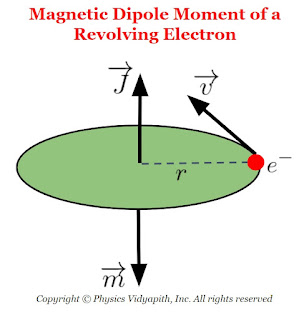### Magnetic dipole moment of a revolving electron

The magnetic dipole moment of a revolving electron (Or Magnetic Moment due to Orbital Angular Momentum):
An electron revolving in an orbit about the nucleus of an atom behaves like a current carrying loop. It is called a minute current-loop and produces a magnetic field. Every current loop is associated with a magnetic moment.Magnetic Dipole Moment of a Revolving Electron

Let us consider, that the magnetic moment associated with a loop carrying current $i$ and having area $A$ is:

$\mu_{L}= i.A \qquad(1)$

The current due to a revolving electron is

$i=\frac{e}{T}$

Where

$T$- The period of revolution of electron motion around the nucleus i.e $T=\frac{2 \pi r}{v}$
$e$- Charge on an electron So from the above equation

$i=\frac{e}{\frac{2 \pi r}{v}}$

$i=\frac{ev}{2 \pi r} \qquad(2)$

The area of the current loop is:

$A=\pi r^{2} \qquad(3)$

Now put the value of $i$ and $A$ in equation $(1)$

$\mu_{L}= \left( \frac{ev}{2 \pi r} \right) \left( \pi r^{2} \right)$

$\mu_{L}= \frac{evr}{2} \qquad(4)$

$\mu_{L}= \left(\frac{evr}{2}\right) \left( \frac{m}{m} \right)$

$\mu_{L}= \left(\frac{e}{2m}\right) \left( mvr \right)$

$\mu_{L}= \left(\frac{e}{2m}\right) L \qquad(5) \qquad (\because L=mvr)$

Where $L$- The orbital angular momentum of the electron and another value of $L$ is

$L=\frac{nh}{2\pi} \qquad(6)$

$\mu_{L}= \left(\frac{e}{2m}\right) \frac{nh}{2\pi}$

 $\mu_{L}= n \: \left(\frac{eh}{4m \pi}\right)$

Where $n=1,2,3......$ is the principle quantum number.

This equation gives the magnetic moment associated with the orbital motion of the electron.

Bohr Magneton:
Bohr Magneton is defined as the angular momentum of an eletcron in ground state.
We know that:
• Principle Quantum Number$(n)=1$
• Charge of a electron $(e)=1.6\times10^{-19} C$
• Planck Constant $(h)= 6.623 \times 10^{-34} J-sec$
• Mass of electron $(m)= 9.1 \times 10^{-31} Kg$

• So the magnetic moment of an electron in the ground state:

$\mu_{B}= n \: \left(\frac{eh}{4m \pi}\right)$

Now subtitute the value of $n,e,h,m$ in above equation:

$\mu_{B}= \frac{1 \times 1.6\times10^{-19} \times 6.623 \times 10^{-34} }{4 \times 3.14 \times 9.1 \times 10^{-31} }$

 $\mu_{B}= 9.274 \times 10^{-24} A-m^{2}$

The magnetic moments due to orbital motion of electrons in higher orbits are multiples of the Bohr magneton value.